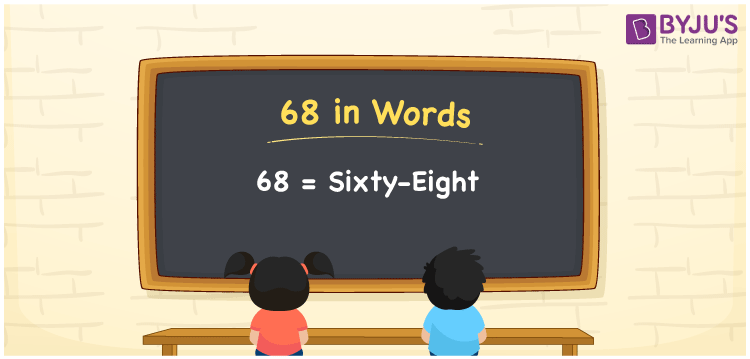# 68 in Words

68 in words is written as “Sixty-eight”. In Mathematics, the number 68 (sixty-eight) is a natural number that is used to represent a value or a quantity. For example, Juhi has Sixty-eight pairs of footwear. Also, we can write Rs.68 as Rupees Sixty-eight. Hence, 68 in words is used in different ways in Maths and in real life. Learn more about Numbers In Words at BYJU’S.

 68 in Words Sixty-eight Sixty-eight in Numbers 68

## 68 in English Words

68 in Words using English alphabets is expressed as given below:## How to Write 68 in Words?

By knowing the place values of each digit in a number, we can name the number or write it in words. To write 68 in words, let us see the positions of each digit in the below table.

 Tens Ones 6 8

From the above table, we can see, 6 is at tens place (60) and 8 is at ones place (8). We need to read the number from right to left, in the table. Thus, together we can write the number, thirty and eight, as Sixty-eight.

### Expanded Form of 68

We can write the expanded form of 68 as:

6 at Tens + 8 at Ones

= 6 × 10 + 8 × 1

= 68

= Sixty-eight

68 is a whole number that is succeeded by 67 and preceded by 69. Find more about the number 68 below:

• 68 in Words – Sixty-eight
• Is 68 an odd number? – No
• Is 68 an even number? – Yes
• Is 68 a perfect square number? – No
• Is 68 a perfect cube number? – No
• Is 68 a prime number? – No
• Is 68 a composite number? – Yes

## Frequently Asked Questions on 68 in words

Q1

### What is 68 in words?

68 in words is given by Sixty-eight.
Q2

### What is the rule to write 68 in words?

68 in words is written based on the place values of each digit. In 68, 6 is at tens place and 8 is at units place. Thus, thirty plus eight is read as Sixty-eight.
Q3

### How to write 68 in English words?

68 in English is written as Sixty-eight